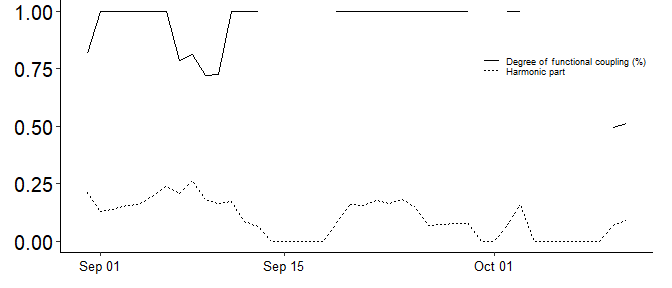# Computing the DFC/HP and manipulating the functions returned objects

#### 2022-11-10

library(digiRhythm)
data <- digiRhythm::df691b_1
data <- resample_dgm(data, 15)
data <- remove_activity_outliers(data)
activity = names(data)
#>  "Steps"

# Degree of Functional Coupling (DFC) and Harmonic Power (HP)

The degree of functional coupling and the harmonic power could be computed using the dfc function as shown below.

The DFC algorithm is a bit complicated. It’s, therefore, needed that we clarify few points about some computing considerations and also about the arguments of the function itself:

• The recommended sampling period is between 15 and 30 min.
• The DFC and HP are computed based on 7 days of data. If the data contains 8 days of data, then we will only have two DFC and HP values. The first value is computed based on Day1-Day7 and the second value is computed based on Day2-Day8. Therefore, the number of DFC and HP data points would be exactly equal: D - 6 (where D is the total number of days).
• Since the DFC is based on the Lomb-Scargle Periodogram (LSP), then the DFC allows having complete missing days in the dataset. In order to compute a DFC for each subset of data, the user needs to split the data into two different subsets beforehand.

The user does not need to worry about looping over days, extract the lomb scargle periodogram for every 7 days and then compile the results to obtain the degree of functional coupling. This is done in loop inside the dfc function. However, there is also a function call lomb_scargle_periodogram where the user can investigate the activity a little bit deeper. We preview the lomb_scargle_periodogram function and its output, then we go to the dfc function.

df <- data[1:672, c('datetime', activity)]
my_lsp <- lomb_scargle_periodogram(df, alpha = 0.01, plot = TRUE)
#> v Correct time format: First column has a POSIXct Format
#> v Number of days: Bigger or equal to 7
#> v Correct numeric format - Column 2 ==> Steps
#> The data is digiRhythm friendly
#> Warning in lomb_scargle_periodogram(df, alpha = 0.01, plot = TRUE): This LSP function is customized to use data with 7 days span only. But, the data contains less or more than 7 days.
#>          A dynamic number of days will be introduced in a later version.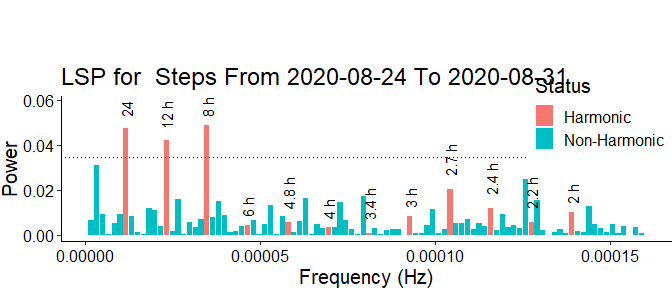In the above plot, we see plenty of information. First, we have the whole periodogram for the dataframe df visualized. Second, we can distinguish between harmonic and non harmonic frequencies. Harmonic frequencies are plotted in blue and tagged with the time period they correspond to. For instance, we can check the power of the 24h cycles, 12h cycles, 6h cycles and so on. Third, we have a dotted horizontal line that makes it easy to visually check which frequencies are considered to originated from noise rather than from a actual signal. Frequency bars that make it above this line are considered to be originated from a real activity. The probability of this event is calculated using the method of Baluev (2008). The plot also shows, as title, the activity time span used for the computation. Warning: At the moment, function only computes the LSP the first 7 days of the data. A loop, to calculate LSP for the whole data, will be included in a later version. However, at the moment user can see the LSP-diagrams of the whole data, if running the dfc function. Before the complete DFC and HP diagramm, each LSP diagramm of the sliding 7-day periods were computed.

Now, to the DFC. The below plot shows the DFC and HP in the same graph. Both variables are percentages.

my_dfc <- dfc(data, activity = activity,  plot = FALSE, verbose = FALSE)
#> v Correct time format: First column has a POSIXct Format
#> v Number of days: Bigger or equal to 7
#> v Correct numeric format - Column 2 ==> Steps
#> The data is digiRhythm friendly
#> Warning in lomb_scargle_periodogram(df_var, alpha = sig, plot = TRUE): This LSP function is customized to use data with 7 days span only. But, the data contains less or more than 7 days.
#>          A dynamic number of days will be introduced in a later version.
#> v Correct time format: First column has a POSIXct Format
#> v Number of days: Bigger or equal to 7
#> v Correct numeric format - Column 2 ==> Steps
#> The data is digiRhythm friendly
#> Warning in lomb_scargle_periodogram(df_var, alpha = sig, plot = TRUE): This LSP function is customized to use data with 7 days span only. But, the data contains less or more than 7 days.
#>          A dynamic number of days will be introduced in a later version.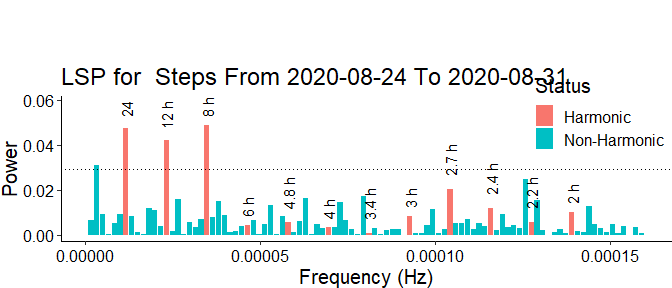#> v Correct time format: First column has a POSIXct Format
#> v Number of days: Bigger or equal to 7
#> v Correct numeric format - Column 2 ==> Steps
#> The data is digiRhythm friendly
#> Warning in lomb_scargle_periodogram(df_var, alpha = sig, plot = TRUE): This LSP function is customized to use data with 7 days span only. But, the data contains less or more than 7 days.
#>          A dynamic number of days will be introduced in a later version.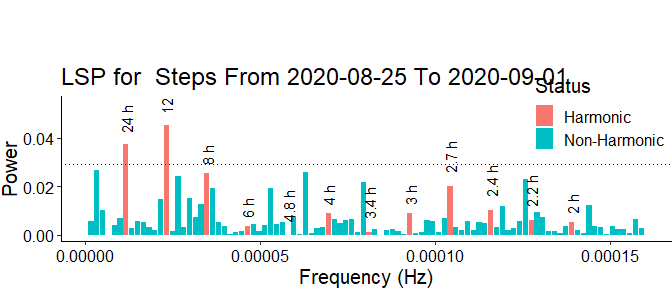#> v Correct time format: First column has a POSIXct Format
#> v Number of days: Bigger or equal to 7
#> v Correct numeric format - Column 2 ==> Steps
#> The data is digiRhythm friendly
#> Warning in lomb_scargle_periodogram(df_var, alpha = sig, plot = TRUE): This LSP function is customized to use data with 7 days span only. But, the data contains less or more than 7 days.
#>          A dynamic number of days will be introduced in a later version.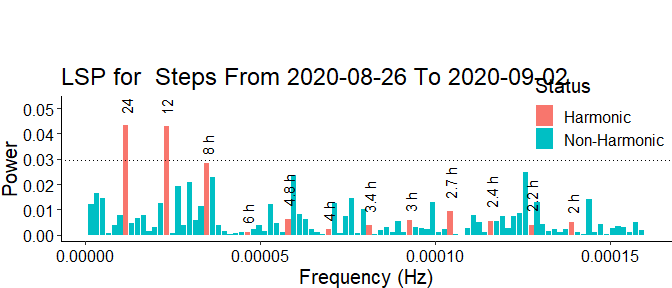#> v Correct time format: First column has a POSIXct Format
#> v Number of days: Bigger or equal to 7
#> v Correct numeric format - Column 2 ==> Steps
#> The data is digiRhythm friendly
#> Warning in lomb_scargle_periodogram(df_var, alpha = sig, plot = TRUE): This LSP function is customized to use data with 7 days span only. But, the data contains less or more than 7 days.
#>          A dynamic number of days will be introduced in a later version.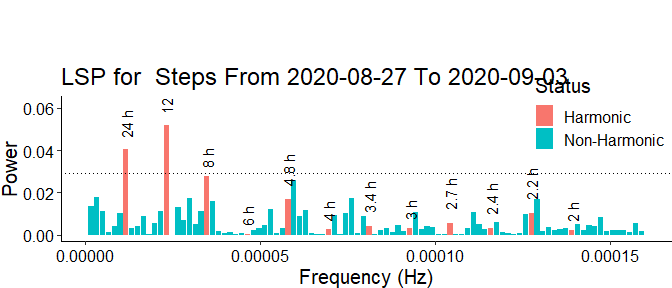#> v Correct time format: First column has a POSIXct Format
#> v Number of days: Bigger or equal to 7
#> v Correct numeric format - Column 2 ==> Steps
#> The data is digiRhythm friendly
#> Warning in lomb_scargle_periodogram(df_var, alpha = sig, plot = TRUE): This LSP function is customized to use data with 7 days span only. But, the data contains less or more than 7 days.
#>          A dynamic number of days will be introduced in a later version.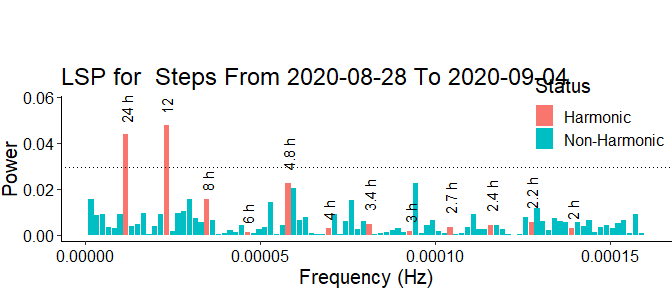#> v Correct time format: First column has a POSIXct Format
#> v Number of days: Bigger or equal to 7
#> v Correct numeric format - Column 2 ==> Steps
#> The data is digiRhythm friendly
#> Warning in lomb_scargle_periodogram(df_var, alpha = sig, plot = TRUE): This LSP function is customized to use data with 7 days span only. But, the data contains less or more than 7 days.
#>          A dynamic number of days will be introduced in a later version.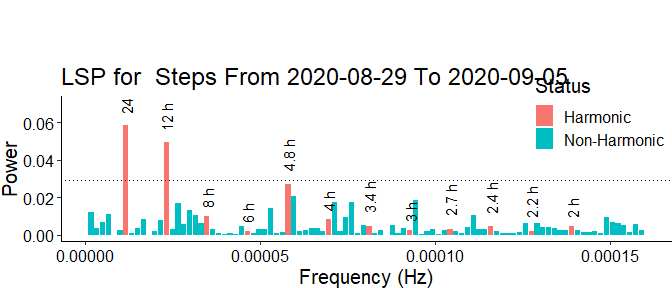#> v Correct time format: First column has a POSIXct Format
#> v Number of days: Bigger or equal to 7
#> v Correct numeric format - Column 2 ==> Steps
#> The data is digiRhythm friendly
#> Warning in lomb_scargle_periodogram(df_var, alpha = sig, plot = TRUE): This LSP function is customized to use data with 7 days span only. But, the data contains less or more than 7 days.
#>          A dynamic number of days will be introduced in a later version.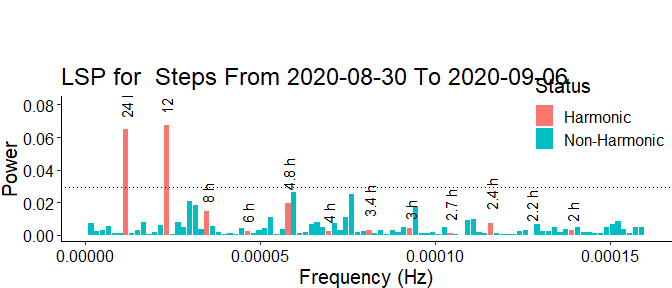#> v Correct time format: First column has a POSIXct Format
#> v Number of days: Bigger or equal to 7
#> v Correct numeric format - Column 2 ==> Steps
#> The data is digiRhythm friendly
#> Warning in lomb_scargle_periodogram(df_var, alpha = sig, plot = TRUE): This LSP function is customized to use data with 7 days span only. But, the data contains less or more than 7 days.
#>          A dynamic number of days will be introduced in a later version.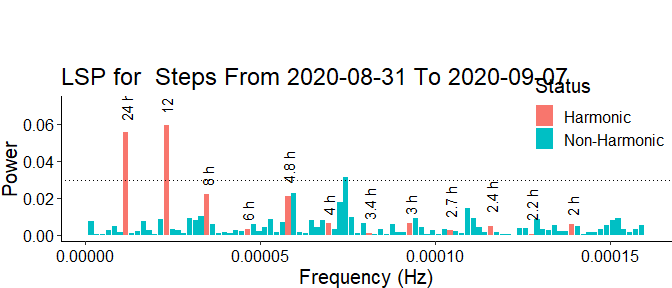#> v Correct time format: First column has a POSIXct Format
#> v Number of days: Bigger or equal to 7
#> v Correct numeric format - Column 2 ==> Steps
#> The data is digiRhythm friendly
#> Warning in lomb_scargle_periodogram(df_var, alpha = sig, plot = TRUE): This LSP function is customized to use data with 7 days span only. But, the data contains less or more than 7 days.
#>          A dynamic number of days will be introduced in a later version.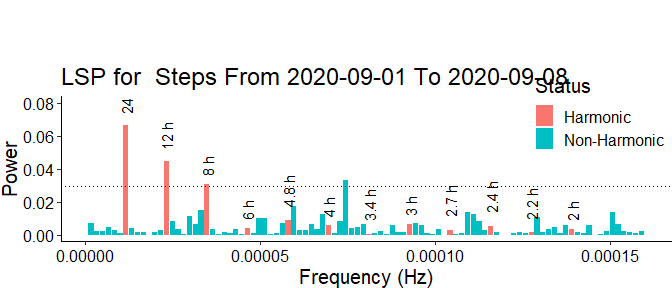#> v Correct time format: First column has a POSIXct Format
#> v Number of days: Bigger or equal to 7
#> v Correct numeric format - Column 2 ==> Steps
#> The data is digiRhythm friendly
#> Warning in lomb_scargle_periodogram(df_var, alpha = sig, plot = TRUE): This LSP function is customized to use data with 7 days span only. But, the data contains less or more than 7 days.
#>          A dynamic number of days will be introduced in a later version.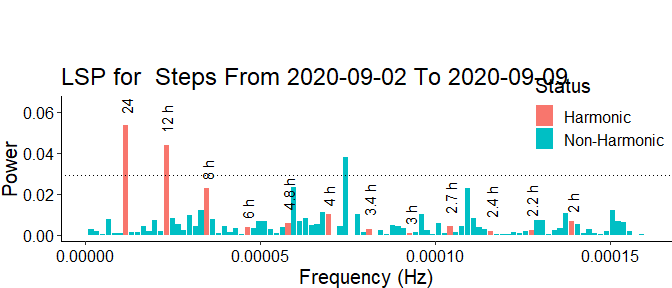#> v Correct time format: First column has a POSIXct Format
#> v Number of days: Bigger or equal to 7
#> v Correct numeric format - Column 2 ==> Steps
#> The data is digiRhythm friendly
#> Warning in lomb_scargle_periodogram(df_var, alpha = sig, plot = TRUE): This LSP function is customized to use data with 7 days span only. But, the data contains less or more than 7 days.
#>          A dynamic number of days will be introduced in a later version.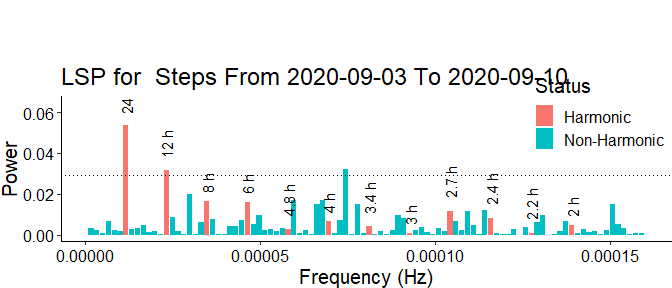#> v Correct time format: First column has a POSIXct Format
#> v Number of days: Bigger or equal to 7
#> v Correct numeric format - Column 2 ==> Steps
#> The data is digiRhythm friendly
#> Warning in lomb_scargle_periodogram(df_var, alpha = sig, plot = TRUE): This LSP function is customized to use data with 7 days span only. But, the data contains less or more than 7 days.
#>          A dynamic number of days will be introduced in a later version.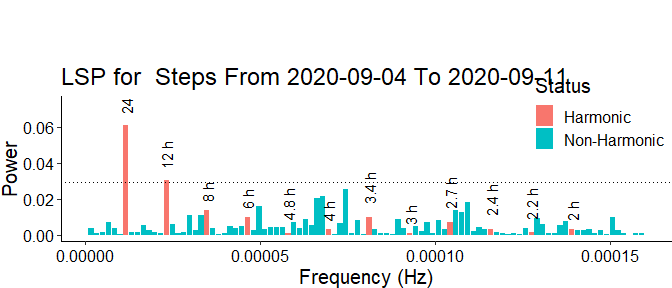#> v Correct time format: First column has a POSIXct Format
#> v Number of days: Bigger or equal to 7
#> v Correct numeric format - Column 2 ==> Steps
#> The data is digiRhythm friendly
#> Warning in lomb_scargle_periodogram(df_var, alpha = sig, plot = TRUE): This LSP function is customized to use data with 7 days span only. But, the data contains less or more than 7 days.
#>          A dynamic number of days will be introduced in a later version.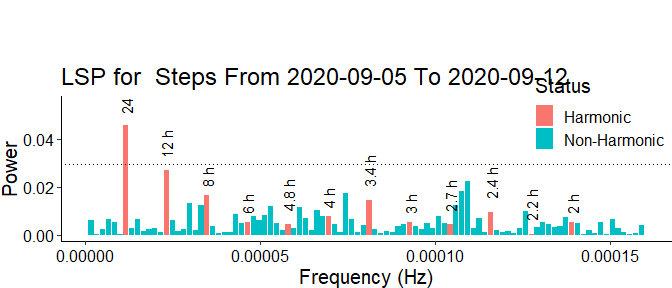#> v Correct time format: First column has a POSIXct Format
#> v Number of days: Bigger or equal to 7
#> v Correct numeric format - Column 2 ==> Steps
#> The data is digiRhythm friendly
#> Warning in lomb_scargle_periodogram(df_var, alpha = sig, plot = TRUE): This LSP function is customized to use data with 7 days span only. But, the data contains less or more than 7 days.
#>          A dynamic number of days will be introduced in a later version.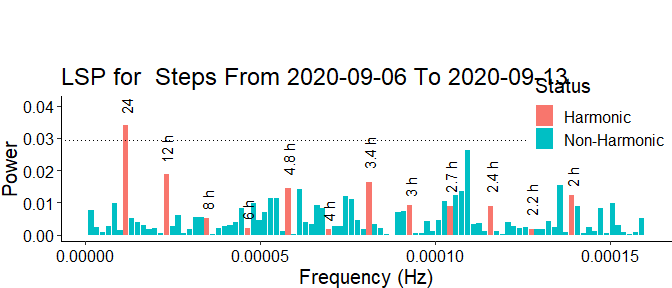#> v Correct time format: First column has a POSIXct Format
#> v Number of days: Bigger or equal to 7
#> v Correct numeric format - Column 2 ==> Steps
#> The data is digiRhythm friendly
#> Warning in lomb_scargle_periodogram(df_var, alpha = sig, plot = TRUE): This LSP function is customized to use data with 7 days span only. But, the data contains less or more than 7 days.
#>          A dynamic number of days will be introduced in a later version.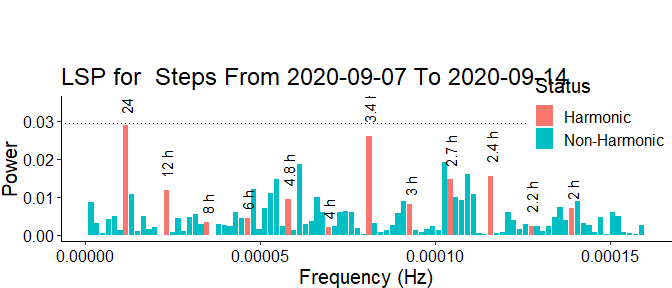#> Warning: Removed 1 rows containing missing values (geom_hline()).
#> v Correct time format: First column has a POSIXct Format
#> v Number of days: Bigger or equal to 7
#> v Correct numeric format - Column 2 ==> Steps
#> The data is digiRhythm friendly
#> Warning in lomb_scargle_periodogram(df_var, alpha = sig, plot = TRUE): This LSP function is customized to use data with 7 days span only. But, the data contains less or more than 7 days.
#>          A dynamic number of days will be introduced in a later version.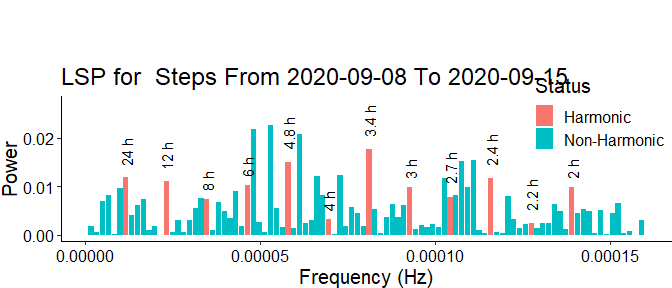#> Warning: Removed 1 rows containing missing values (geom_hline()).
#> v Correct time format: First column has a POSIXct Format
#> v Number of days: Bigger or equal to 7
#> v Correct numeric format - Column 2 ==> Steps
#> The data is digiRhythm friendly
#> Warning in lomb_scargle_periodogram(df_var, alpha = sig, plot = TRUE): This LSP function is customized to use data with 7 days span only. But, the data contains less or more than 7 days.
#>          A dynamic number of days will be introduced in a later version.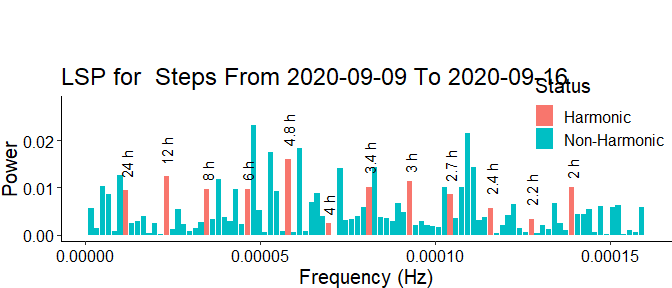#> Warning: Removed 1 rows containing missing values (geom_hline()).
#> v Correct time format: First column has a POSIXct Format
#> v Number of days: Bigger or equal to 7
#> v Correct numeric format - Column 2 ==> Steps
#> The data is digiRhythm friendly
#> Warning in lomb_scargle_periodogram(df_var, alpha = sig, plot = TRUE): This LSP function is customized to use data with 7 days span only. But, the data contains less or more than 7 days.
#>          A dynamic number of days will be introduced in a later version.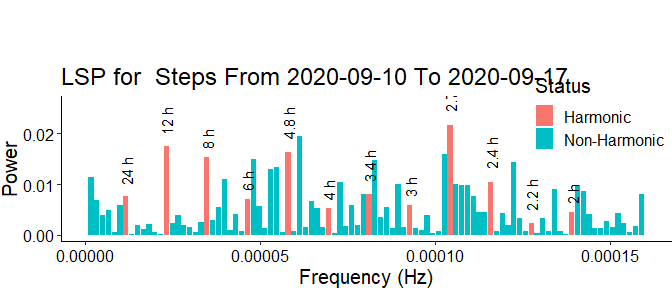#> Warning: Removed 1 rows containing missing values (geom_hline()).
#> v Correct time format: First column has a POSIXct Format
#> v Number of days: Bigger or equal to 7
#> v Correct numeric format - Column 2 ==> Steps
#> The data is digiRhythm friendly
#> Warning in lomb_scargle_periodogram(df_var, alpha = sig, plot = TRUE): This LSP function is customized to use data with 7 days span only. But, the data contains less or more than 7 days.
#>          A dynamic number of days will be introduced in a later version.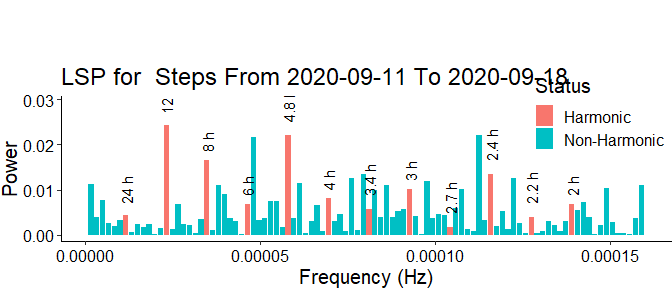#> v Correct time format: First column has a POSIXct Format
#> v Number of days: Bigger or equal to 7
#> v Correct numeric format - Column 2 ==> Steps
#> The data is digiRhythm friendly
#> Warning in lomb_scargle_periodogram(df_var, alpha = sig, plot = TRUE): This LSP function is customized to use data with 7 days span only. But, the data contains less or more than 7 days.
#>          A dynamic number of days will be introduced in a later version.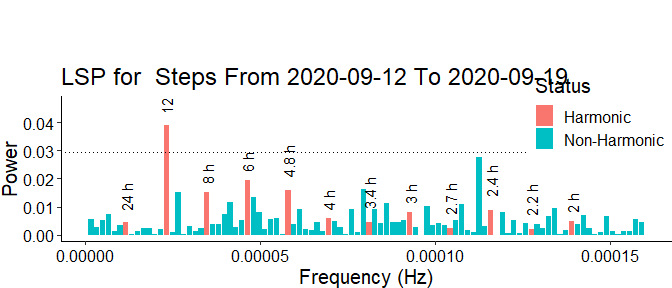#> v Correct time format: First column has a POSIXct Format
#> v Number of days: Bigger or equal to 7
#> v Correct numeric format - Column 2 ==> Steps
#> The data is digiRhythm friendly
#> Warning in lomb_scargle_periodogram(df_var, alpha = sig, plot = TRUE): This LSP function is customized to use data with 7 days span only. But, the data contains less or more than 7 days.
#>          A dynamic number of days will be introduced in a later version.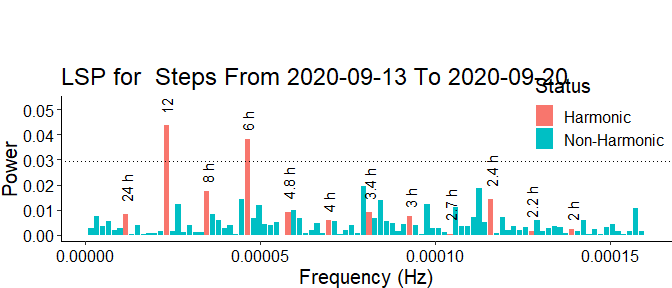#> v Correct time format: First column has a POSIXct Format
#> v Number of days: Bigger or equal to 7
#> v Correct numeric format - Column 2 ==> Steps
#> The data is digiRhythm friendly
#> Warning in lomb_scargle_periodogram(df_var, alpha = sig, plot = TRUE): This LSP function is customized to use data with 7 days span only. But, the data contains less or more than 7 days.
#>          A dynamic number of days will be introduced in a later version.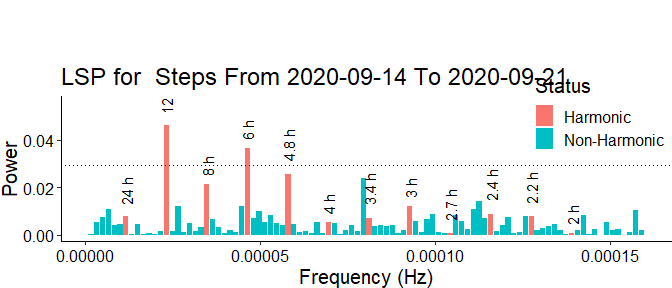#> v Correct time format: First column has a POSIXct Format
#> v Number of days: Bigger or equal to 7
#> v Correct numeric format - Column 2 ==> Steps
#> The data is digiRhythm friendly
#> Warning in lomb_scargle_periodogram(df_var, alpha = sig, plot = TRUE): This LSP function is customized to use data with 7 days span only. But, the data contains less or more than 7 days.
#>          A dynamic number of days will be introduced in a later version.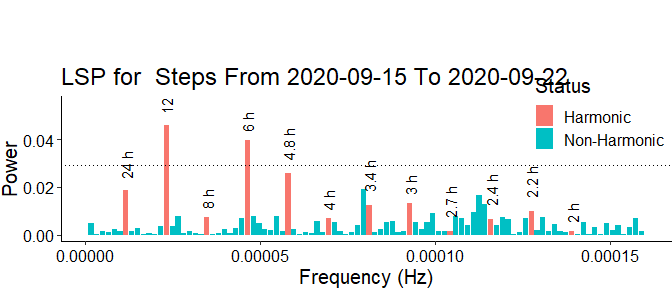#> v Correct time format: First column has a POSIXct Format
#> v Number of days: Bigger or equal to 7
#> v Correct numeric format - Column 2 ==> Steps
#> The data is digiRhythm friendly
#> Warning in lomb_scargle_periodogram(df_var, alpha = sig, plot = TRUE): This LSP function is customized to use data with 7 days span only. But, the data contains less or more than 7 days.
#>          A dynamic number of days will be introduced in a later version.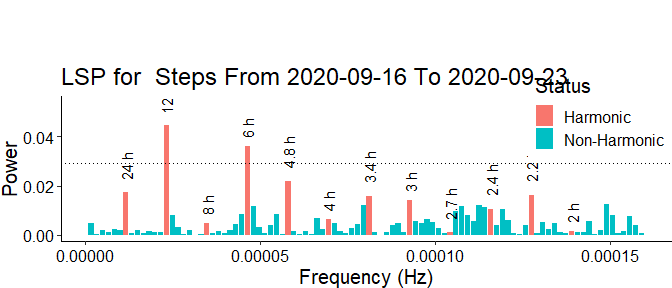#> v Correct time format: First column has a POSIXct Format
#> v Number of days: Bigger or equal to 7
#> v Correct numeric format - Column 2 ==> Steps
#> The data is digiRhythm friendly
#> Warning in lomb_scargle_periodogram(df_var, alpha = sig, plot = TRUE): This LSP function is customized to use data with 7 days span only. But, the data contains less or more than 7 days.
#>          A dynamic number of days will be introduced in a later version.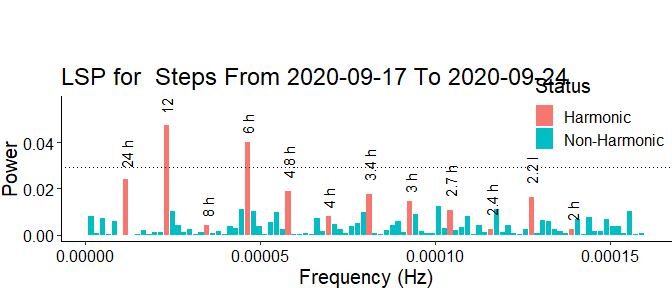#> v Correct time format: First column has a POSIXct Format
#> v Number of days: Bigger or equal to 7
#> v Correct numeric format - Column 2 ==> Steps
#> The data is digiRhythm friendly
#> Warning in lomb_scargle_periodogram(df_var, alpha = sig, plot = TRUE): This LSP function is customized to use data with 7 days span only. But, the data contains less or more than 7 days.
#>          A dynamic number of days will be introduced in a later version.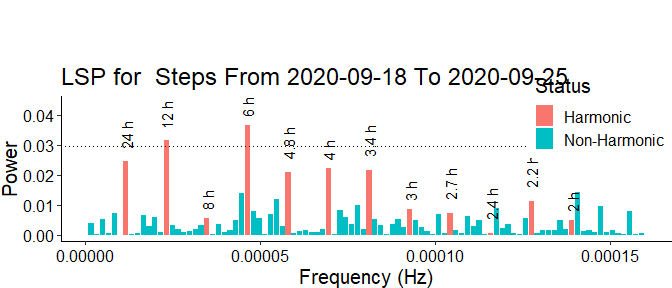#> v Correct time format: First column has a POSIXct Format
#> v Number of days: Bigger or equal to 7
#> v Correct numeric format - Column 2 ==> Steps
#> The data is digiRhythm friendly
#> Warning in lomb_scargle_periodogram(df_var, alpha = sig, plot = TRUE): This LSP function is customized to use data with 7 days span only. But, the data contains less or more than 7 days.
#>          A dynamic number of days will be introduced in a later version.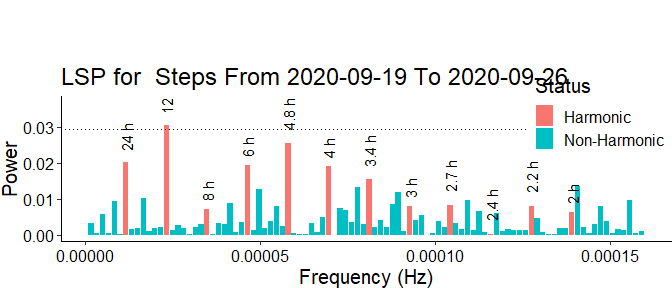#> v Correct time format: First column has a POSIXct Format
#> v Number of days: Bigger or equal to 7
#> v Correct numeric format - Column 2 ==> Steps
#> The data is digiRhythm friendly
#> Warning in lomb_scargle_periodogram(df_var, alpha = sig, plot = TRUE): This LSP function is customized to use data with 7 days span only. But, the data contains less or more than 7 days.
#>          A dynamic number of days will be introduced in a later version.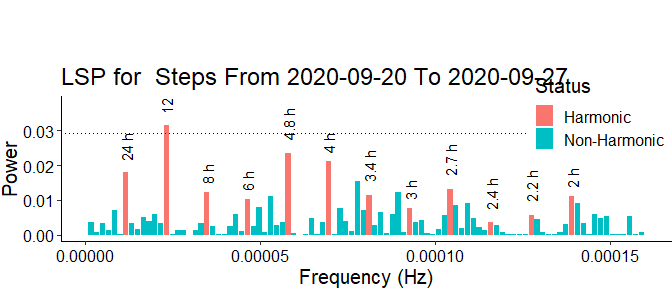#> v Correct time format: First column has a POSIXct Format
#> v Number of days: Bigger or equal to 7
#> v Correct numeric format - Column 2 ==> Steps
#> The data is digiRhythm friendly
#> Warning in lomb_scargle_periodogram(df_var, alpha = sig, plot = TRUE): This LSP function is customized to use data with 7 days span only. But, the data contains less or more than 7 days.
#>          A dynamic number of days will be introduced in a later version.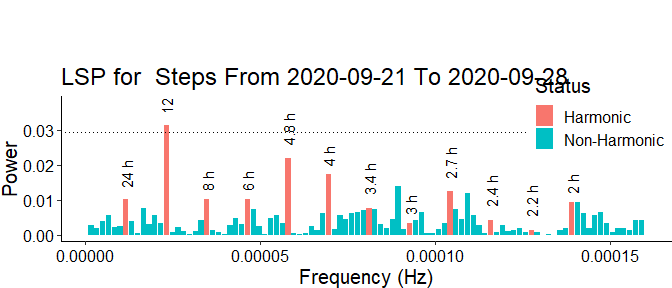#> v Correct time format: First column has a POSIXct Format
#> v Number of days: Bigger or equal to 7
#> v Correct numeric format - Column 2 ==> Steps
#> The data is digiRhythm friendly
#> Warning in lomb_scargle_periodogram(df_var, alpha = sig, plot = TRUE): This LSP function is customized to use data with 7 days span only. But, the data contains less or more than 7 days.
#>          A dynamic number of days will be introduced in a later version.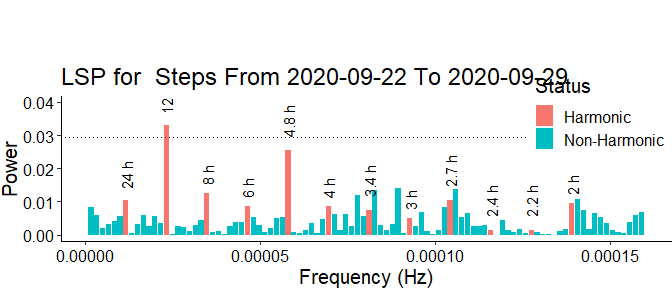#> v Correct time format: First column has a POSIXct Format
#> v Number of days: Bigger or equal to 7
#> v Correct numeric format - Column 2 ==> Steps
#> The data is digiRhythm friendly
#> Warning in lomb_scargle_periodogram(df_var, alpha = sig, plot = TRUE): This LSP function is customized to use data with 7 days span only. But, the data contains less or more than 7 days.
#>          A dynamic number of days will be introduced in a later version.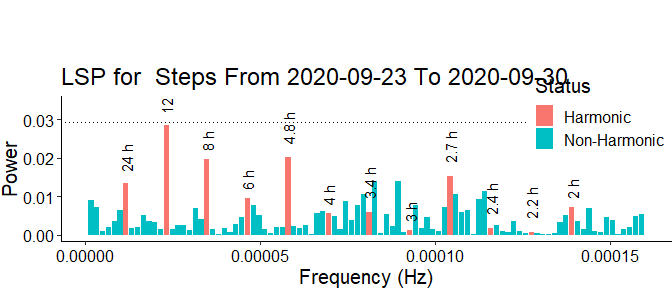#> Warning: Removed 1 rows containing missing values (geom_hline()).
#> v Correct time format: First column has a POSIXct Format
#> v Number of days: Bigger or equal to 7
#> v Correct numeric format - Column 2 ==> Steps
#> The data is digiRhythm friendly
#> Warning in lomb_scargle_periodogram(df_var, alpha = sig, plot = TRUE): This LSP function is customized to use data with 7 days span only. But, the data contains less or more than 7 days.
#>          A dynamic number of days will be introduced in a later version.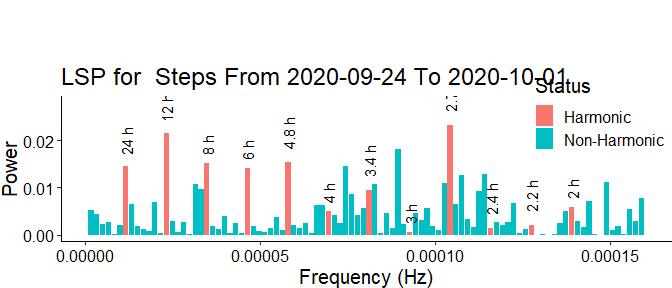#> v Correct time format: First column has a POSIXct Format
#> v Number of days: Bigger or equal to 7
#> v Correct numeric format - Column 2 ==> Steps
#> The data is digiRhythm friendly
#> Warning in lomb_scargle_periodogram(df_var, alpha = sig, plot = TRUE): This LSP function is customized to use data with 7 days span only. But, the data contains less or more than 7 days.
#>          A dynamic number of days will be introduced in a later version.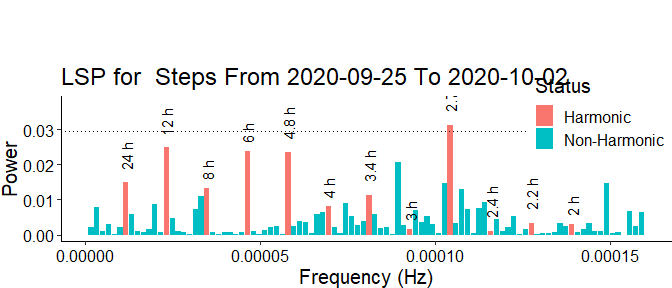#> v Correct time format: First column has a POSIXct Format
#> v Number of days: Bigger or equal to 7
#> v Correct numeric format - Column 2 ==> Steps
#> The data is digiRhythm friendly
#> Warning in lomb_scargle_periodogram(df_var, alpha = sig, plot = TRUE): This LSP function is customized to use data with 7 days span only. But, the data contains less or more than 7 days.
#>          A dynamic number of days will be introduced in a later version.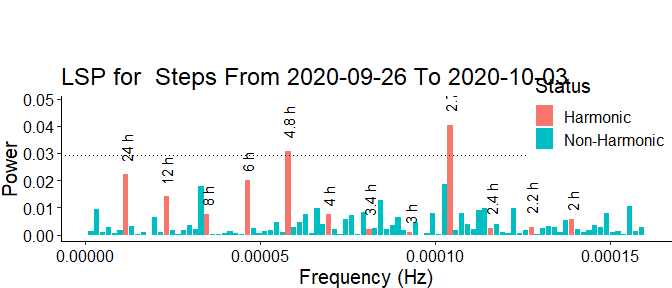#> v Correct time format: First column has a POSIXct Format
#> v Number of days: Bigger or equal to 7
#> v Correct numeric format - Column 2 ==> Steps
#> The data is digiRhythm friendly
#> Warning in lomb_scargle_periodogram(df_var, alpha = sig, plot = TRUE): This LSP function is customized to use data with 7 days span only. But, the data contains less or more than 7 days.
#>          A dynamic number of days will be introduced in a later version.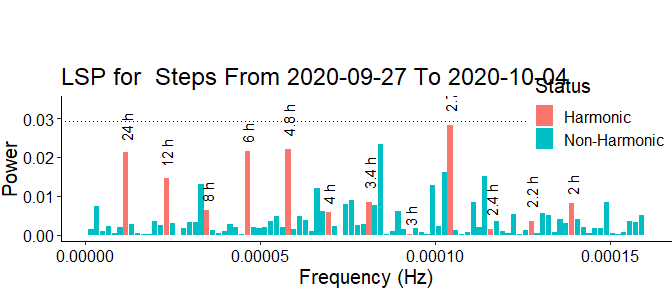#> v Correct time format: First column has a POSIXct Format
#> v Number of days: Bigger or equal to 7
#> v Correct numeric format - Column 2 ==> Steps
#> The data is digiRhythm friendly
#> Warning in lomb_scargle_periodogram(df_var, alpha = sig, plot = TRUE): This LSP function is customized to use data with 7 days span only. But, the data contains less or more than 7 days.
#>          A dynamic number of days will be introduced in a later version.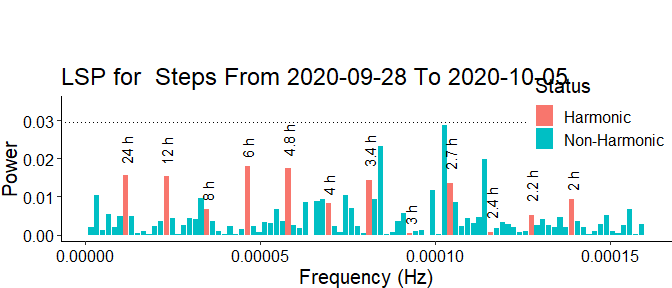#> Warning: Removed 1 rows containing missing values (geom_hline()).
#> v Correct time format: First column has a POSIXct Format
#> v Number of days: Bigger or equal to 7
#> v Correct numeric format - Column 2 ==> Steps
#> The data is digiRhythm friendly
#> Warning in lomb_scargle_periodogram(df_var, alpha = sig, plot = TRUE): This LSP function is customized to use data with 7 days span only. But, the data contains less or more than 7 days.
#>          A dynamic number of days will be introduced in a later version.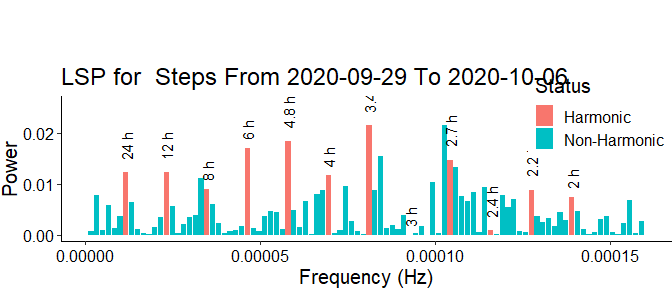#> Warning: Removed 1 rows containing missing values (geom_hline()).
#> v Correct time format: First column has a POSIXct Format
#> v Number of days: Bigger or equal to 7
#> v Correct numeric format - Column 2 ==> Steps
#> The data is digiRhythm friendly
#> Warning in lomb_scargle_periodogram(df_var, alpha = sig, plot = TRUE): This LSP function is customized to use data with 7 days span only. But, the data contains less or more than 7 days.
#>          A dynamic number of days will be introduced in a later version.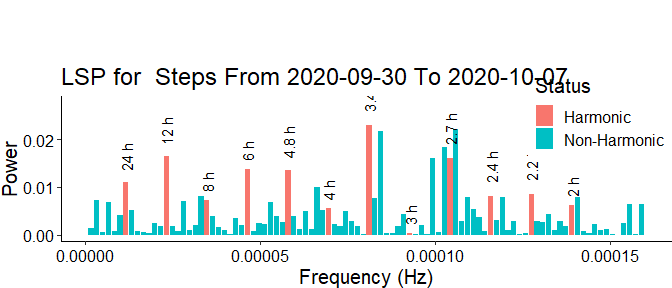#> Warning: Removed 1 rows containing missing values (geom_hline()).
#> v Correct time format: First column has a POSIXct Format
#> v Number of days: Bigger or equal to 7
#> v Correct numeric format - Column 2 ==> Steps
#> The data is digiRhythm friendly
#> Warning in lomb_scargle_periodogram(df_var, alpha = sig, plot = TRUE): This LSP function is customized to use data with 7 days span only. But, the data contains less or more than 7 days.
#>          A dynamic number of days will be introduced in a later version.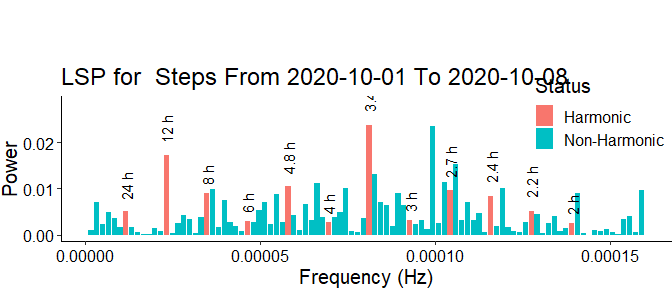#> Warning: Removed 1 rows containing missing values (geom_hline()).
#> v Correct time format: First column has a POSIXct Format
#> v Number of days: Bigger or equal to 7
#> v Correct numeric format - Column 2 ==> Steps
#> The data is digiRhythm friendly
#> Warning in lomb_scargle_periodogram(df_var, alpha = sig, plot = TRUE): This LSP function is customized to use data with 7 days span only. But, the data contains less or more than 7 days.
#>          A dynamic number of days will be introduced in a later version.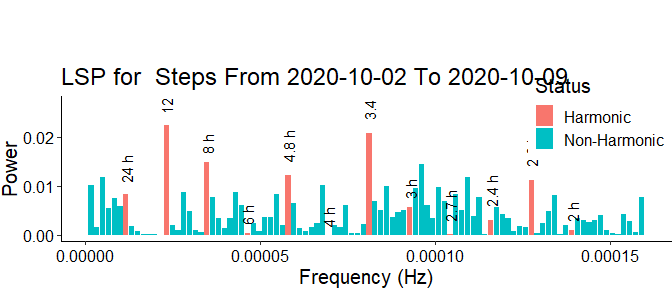#> v Correct time format: First column has a POSIXct Format
#> v Number of days: Bigger or equal to 7
#> v Correct numeric format - Column 2 ==> Steps
#> The data is digiRhythm friendly
#> Warning in lomb_scargle_periodogram(df_var, alpha = sig, plot = TRUE): This LSP function is customized to use data with 7 days span only. But, the data contains less or more than 7 days.
#>          A dynamic number of days will be introduced in a later version.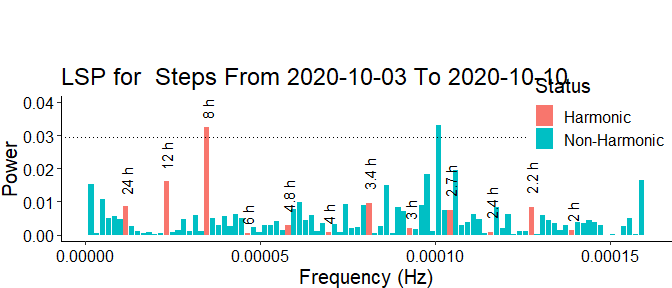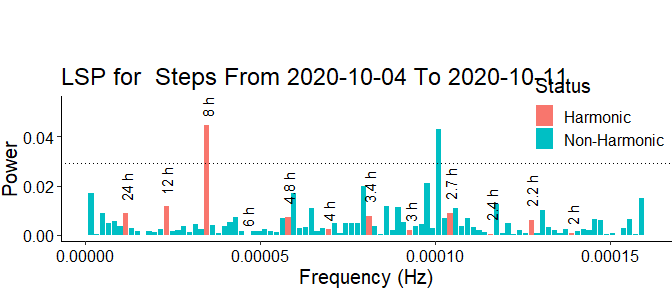#Setting plot to TRUE will visualize all the LSP plots (like the one above) during each sliding window. Rather used for deeper investigation and debugging.
print(my_dfc)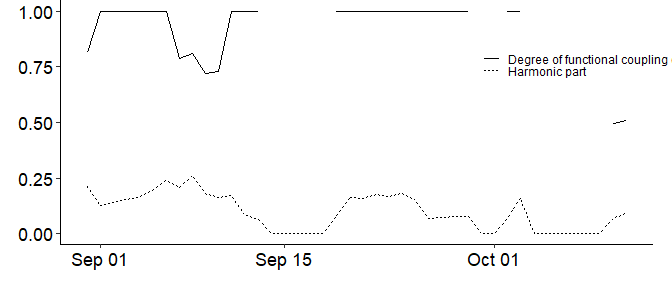# Accessing output data and changing the plots using DigiRhythm

The DFC function returns a object of type ggplot:

class(my_dfc)
#>  "gg"     "ggplot"

As shown, the class is gg or ggplot. This allows the user to: - Access the data of DFC or HP directly from the returned object. - Add aesthetics layer to the plot as needed.

To access the data, user has to use the data component of the DFC object. We illustrate this in the below code:

head(my_dfc$data) #> from to dfc hp #> 1 2020-08-25 2020-08-31 0.8159025 0.2107367 #> 2 2020-08-26 2020-09-01 1.0000000 0.1283563 #> 3 2020-08-27 2020-09-02 1.0000000 0.1379133 #> 4 2020-08-28 2020-09-03 1.0000000 0.1539973 #> 5 2020-08-29 2020-09-04 1.0000000 0.1620310 #> 6 2020-08-30 2020-09-05 1.0000000 0.1951330 As shown, we can access a dataframe where the first comlumn is the date and the second two columns are the DFC and HP respectively. This will allow the users to be able to use the returned data in further studies such as modelling. Users can also change the aesthetics of the plot directly if they wish. This is possible by adding a ggplot layer to the returned DFC object. For instance, this is the original plot returned by the DFC: print(my_dfc)As an illustrative example, in the below code we add a new aesthetic layer to the ggplot object. This layer does nothing but increasing the width of the x-axis. Users can add any other aesthetic layer as they wish; the immagination is the only limit. library(ggplot2) new_plot <- my_dfc + theme( text=element_text(family="Times", size=8), axis.text.y=element_text(size=15, colour="black")) print(new_plot) #> Warning in grid.Call(C_stringMetric, as.graphicsAnnot(x$label)): font family not
#> found in Windows font database

#> Warning in grid.Call(C_stringMetric, as.graphicsAnnot(x$label)): font family not #> found in Windows font database #> Warning in grid.Call(C_stringMetric, as.graphicsAnnot(x$label)): font family not
#> found in Windows font database

#> Warning in grid.Call(C_stringMetric, as.graphicsAnnot(x$label)): font family not #> found in Windows font database #> Warning in grid.Call(C_textBounds, as.graphicsAnnot(x$label), x$x, x$y, : font

#> Warning in grid.Call(C_textBounds, as.graphicsAnnot(x$label), x$x, x$y, : font #> family not found in Windows font database #> Warning in grid.Call(C_textBounds, as.graphicsAnnot(x$label), x$x, x$y, : font

#> Warning in grid.Call(C_textBounds, as.graphicsAnnot(x$label), x$x, x$y, : font #> family not found in Windows font database #> Warning in grid.Call(C_textBounds, as.graphicsAnnot(x$label), x$x, x$y, : font
#> Warning in grid.Call(C_textBounds, as.graphicsAnnot(x$label), x$x, x$y, : font #> family not found in Windows font database #> Warning in grid.Call(C_textBounds, as.graphicsAnnot(x$label), x$x, x$y, : font
#> Warning in grid.Call(C_textBounds, as.graphicsAnnot(x$label), x$x, x$y, : font #> family not found in Windows font database #> Warning in grid.Call(C_textBounds, as.graphicsAnnot(x$label), x$x, x$y, : font
#> Warning in grid.Call(C_textBounds, as.graphicsAnnot(x$label), x$x, x\$y, : font
#> family not found in Windows font database